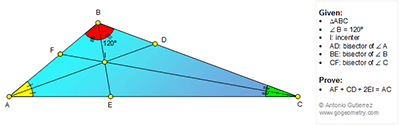## Friday, January 6, 2017

### Geometry Problem 1304: Triangle, 120 Degrees, Angle Bisector, Incenter, Measurement

Geometry Problem. Post your solution in the comment box below.
Level: Mathematics Education, High School, Honors Geometry, College.

Details: Click on the figure below.1.https://goo.gl/photos/dB3gitFmAEA66Z6UA

Let EF cut I at J and EP//AB ( P on BC) See sketch
We have ∠ABE=∠EBD=∠BEP=60 => BEP is isosceles=> BE=BP
Since CF bisect angle ACB => AF/FB=AC/BC
But AC/BC=AE/BP ( Thales’s theorem)= AE/BE
So EF bisect angle AEB
Per the result of problem 1303 with triangle ABE ( AI and EF bisect angles A and E and angle B= 60)
We have AF+IE= AE … (1)
Similarly with triangle EBC we have IE+DC=EC.. (2)
Add (1) to (2) we have AF+2.EI+DC=AE+EC=AC

2.https://goo.gl/photos/REErnXGV8nZiPM4F7
(new improved solution)
Draw EP//BC
Locate points M and N on AC such that AF=AM and CN=CD ( see sketch)
We have ∠AIC= 90+1/2.B= 150
∠DIC=∠CIN=∠AIM=∠AIF=30 => ∠MIN=90
Triangle BEP is equilateral
AD bisect angle A => BD/DC=AB/AC=BP/EC=BE/EC …( Thales’s theorem)
So ED bisect angle BEC => E is the circumcenter of right triangle MIN
AC=AM+MN+NC=AF+2.EI+ CD

3.U need not be Einstein to figure out that < AIC = 150.

Let X, Y be on AC such that AF = AX & CD=CY.

Tr.s AFI & AXI are congruent SAS and so
< AIF = < AIX = 30.
Similarly < CIY = 30 and so < XIY = 90.
Further < FIB = 90-A/2 (considering angles of Tr. AIB) = <CIE, hence < EIY = 60-A/2 and so < EIX = A/2 + 30
But < EXI = A/2 + 30 (external angle of Tr. AIX)
So E is the Centre of right triangle XIE

Therefore AF + CD + 2IE = AX + CY + XY = AC

Sumith Peiris
Moratuwa
Sri Lanka

4.Problem 1304
Let's say that the EF and AD meet at I1 , CF and ED meet at I2.Then the BD is bisector
external angle <ABE so the point D is A-excenter of the triangle ABE(<ABE=60=<EBC).Then the ED is bisector of the <BEC.Similar the FE is bisector of the <AEB.So I1 is incenter of the triangle ABE and I2 is incenter of the triangle BEC.Therefore from problem 1303 we have ΑF+2EI+CD=(AF+IE)+(IE+DC)=AE+EC=AC.
APOSTOLIS MANOLOUDIS KORYDALLOS PIRAEUS GREECE

1.Good shortcut Apostolis

2.Sumith thank you very much.